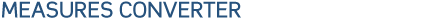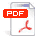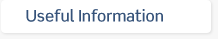#Temperature

 º F 32 40 50 60 70 75 085 95 105 140 175 212 º C 0 4,4 10 15,6 21,1 23,9 29,4 35 40,6 60 79,4 100

To convert Celsius to Fahrenheit multiply by 9, divide by 5 and add 32
To convert Fahrenheit into Celsius subtract 32, multiply by 5 and devide by 9

Speed

 mph 20 30 40 50 60 70 80 90 100 km/h 32 48 64 80 96 112 128 144 160

To convert milles into Kms. Multiply by 1.609
To convert Kms into milles by 0.6214

Other convertion measures

 ton long (t or tn) 1016 1016 kg 1.016e+06 g Mass_Weight ton metric (t or tn) 1000 1000 kg 1e+06 g Mass_Weight ton short (t) 907.2 907.2 kg 907200 g Mass_Weight yard (yd) 0.9144 0.9144 m 91.44 cm Distance_Length

 square centimeter (cm2) cm*cm 0.0001 m2 1 cm2 Area square foot (f2) f*f 0.092903 m2 929.03 cm2 Area square inch (in2) 0.092903 m2 6.4516 cm2 Area

#### MAS INFORMACIONTable for diferent measure, weight, volume and size Download pdf